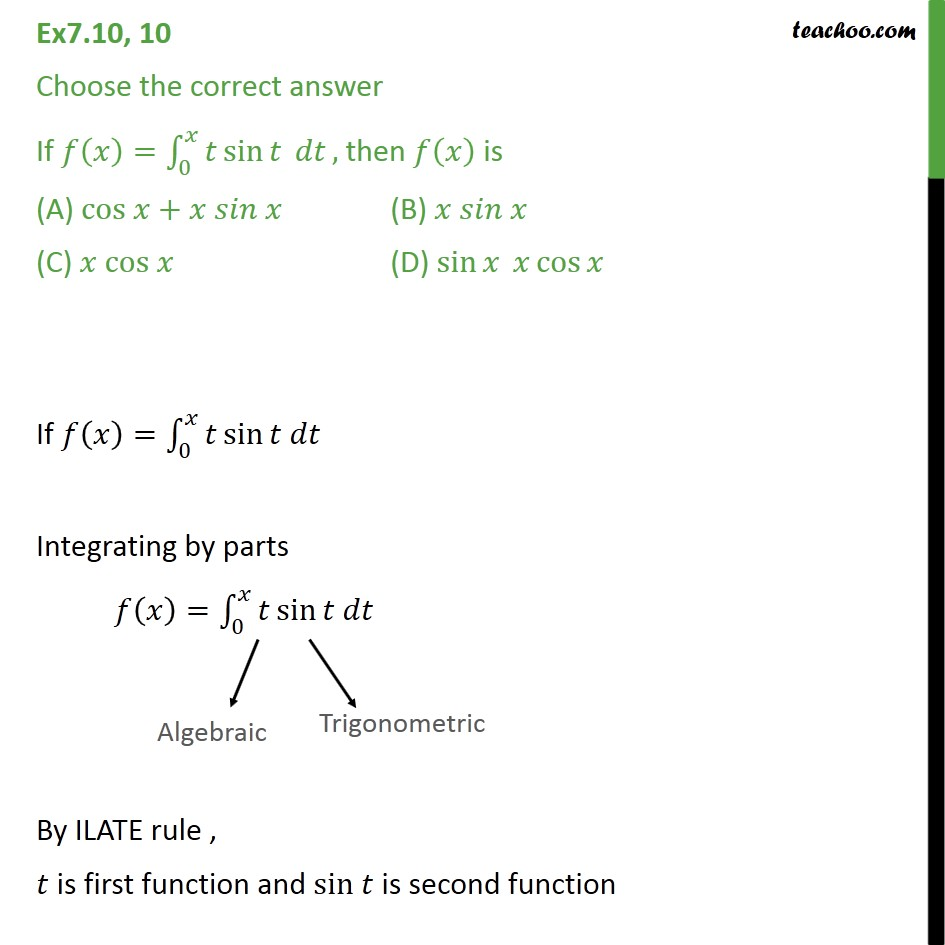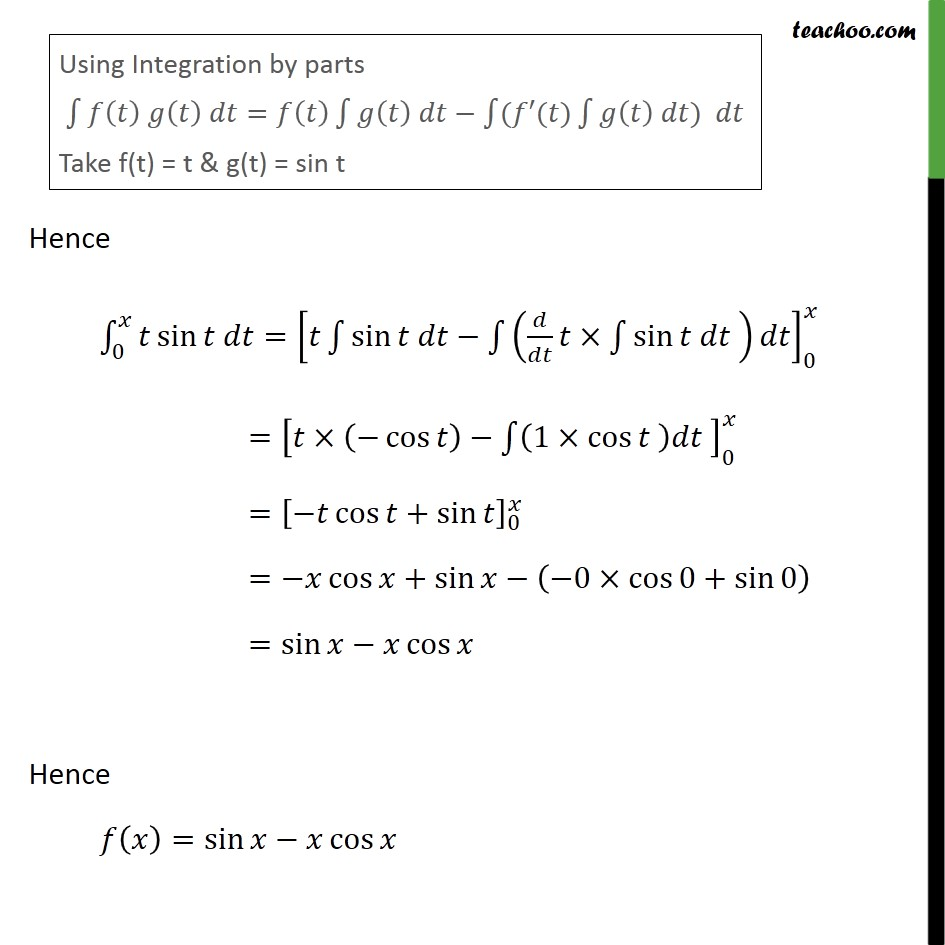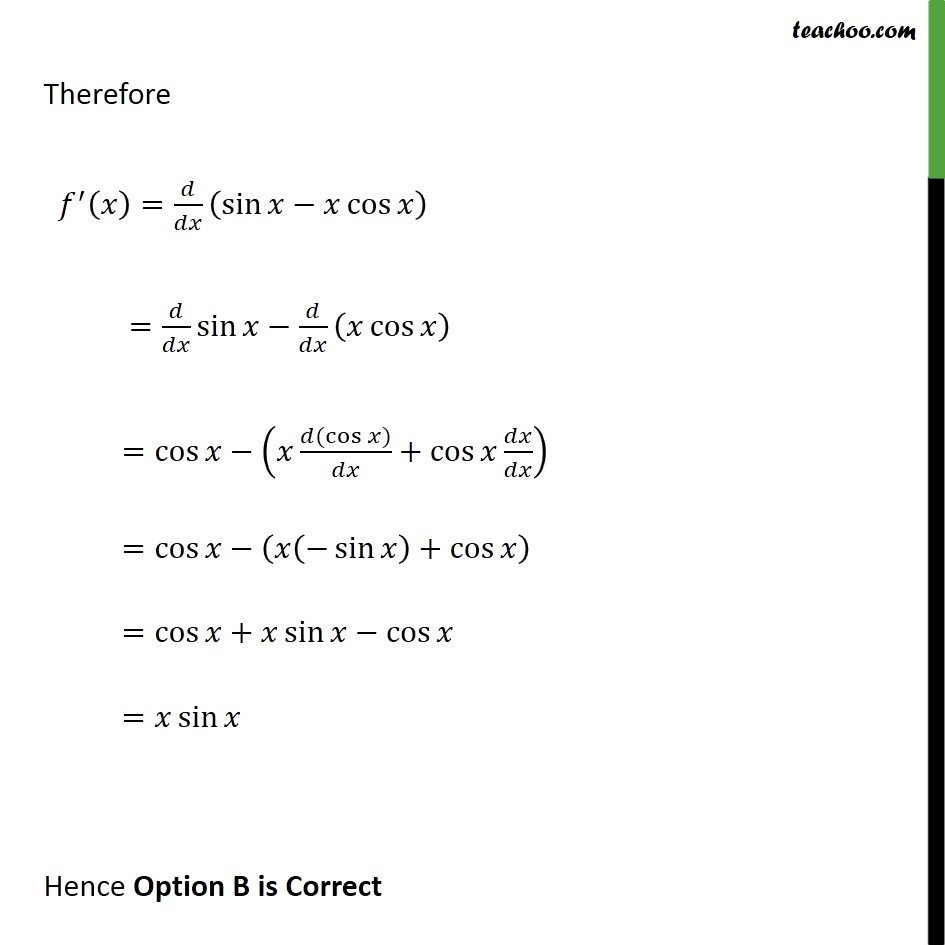1. Chapter 7 Class 12 Integrals
2. Concept wise
3. Definite Integration - By Formulae

Transcript

Ex7.10, 10 Choose the correct answer If 𝑓 𝑥﷯= 0﷮𝑥﷮𝑡 sin﷮𝑡﷯ 𝑑𝑡﷯, then 𝑓 𝑥﷯ is (A) cos 𝑥+𝑥 𝑠𝑖𝑛 𝑥 (B) 𝑥 𝑠𝑖𝑛 𝑥 (C) 𝑥 cos 𝑥 (D) sin﷮𝑥﷯ 𝑥 cos﷮𝑥﷯ If 𝑓 𝑥﷯= 0﷮𝑥﷮𝑡 sin﷮𝑡 𝑑𝑡﷯﷯ Integrating by parts 𝑓 𝑥﷯= 0﷮𝑥﷮𝑡 sin﷮𝑡 𝑑𝑡﷯﷯ By ILATE rule , 𝑡 is first function and sin 𝑡 is second function Hence 0﷮𝑥﷮𝑡 sin﷮𝑡 𝑑𝑡= 𝑡 ﷮﷮ sin﷮𝑡 𝑑𝑡− ﷮﷮ 𝑑﷮𝑑𝑡﷯𝑡× ﷮﷮ sin﷮𝑡 𝑑𝑡﷯﷯ ﷯𝑑𝑡﷯﷯﷯﷯﷮0﷮𝑥﷯﷯﷯ = 𝑡× − cos﷮𝑡﷯﷯− ﷮﷮ 1× cos﷮𝑡﷯ ﷯𝑑𝑡﷯ ﷯﷮0﷮𝑥﷯ = −𝑡 cos﷮𝑡+ sin﷮𝑡﷯﷯﷯﷮0﷮𝑥﷯ =−𝑥 cos﷮𝑥+ sin﷮𝑥− −0× cos﷮0+ sin﷮0﷯﷯﷯﷯﷯ = sin﷮𝑥﷯−𝑥 cos﷮𝑥﷯ Hence 𝑓 𝑥﷯= sin﷮𝑥−𝑥 cos﷮𝑥﷯﷯ Therefore 𝑓﷮′﷯ 𝑥﷯= 𝑑﷮𝑑𝑥﷯ sin﷮𝑥−𝑥 cos﷮𝑥﷯﷯﷯ = 𝑑﷮𝑑𝑥﷯ sin﷮𝑥− 𝑑﷮𝑑𝑥﷯ 𝑥 cos﷮𝑥﷯﷯﷯ = cos﷮𝑥− 𝑥 𝑑( cos﷮𝑥﷯)﷮𝑑𝑥﷯+ cos﷮𝑥 𝑑𝑥﷮𝑑𝑥﷯﷯﷯﷯ = cos﷮𝑥− 𝑥 − sin﷮𝑥﷯﷯+ cos﷮𝑥﷯﷯﷯ = cos﷮𝑥+𝑥 sin﷮𝑥− cos﷮𝑥﷯﷯﷯ =𝑥 sin﷮𝑥﷯ Hence Option B is Correct

Definite Integration - By Formulae